## Golden Mean Ratio: Egyptian Sculpture

Math in Ancient Sculpture is one of the most interesting and intriguing applications of mathematics, both formula equation and geometric structure.  This article examines the Golden Mean Ratio and Fibonacci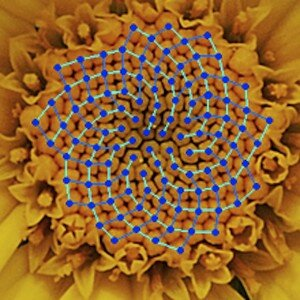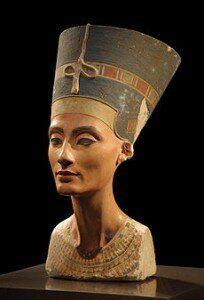Sequence found in the Bust of Nefertiti in Egypt in the early 1900’s. Nefertiti literally, means, “the beautiful one has come”, and is the 14th-century BC Great Royal Wife (chief co-regent) of the Egyptian Pharaoh Akhenaten of the Eighteenth dynasty of Egypt, 1352 BC to 1336 BC.  The iconic Bust of Nefertiti is in Neues Museum of Berlin; though originally in Egypt (En.   Wikipedia.   Org / wiki/Nefertiti_Bust   (230px-Nofretete_Neues_Museum 2 .jpg)  During excavation of Nefertit’s Bust, one of the items found noted Thutmose as being the court sculptor of Egyptian Pharaoh Akhenaten, Nefertiti’s Royal Husband.  In Mathematics of Harmony: From Euclid to Contemporary Mathematics and Computer Science, Stakho speaks of the Berlusian philosopher Edward Soroko’s attempts to determine what mathematical ideas were used in the creation of Her Bust, “…Harmony was the perogative of the Divine order that dominated the universe, and geometry was the main tool of its expression.

Nefertiti, playing role of Goddess, thus Her image personifying the Wisdom of the world, must have been formed with geometrical perfection and irreproachable harmony, beauty and clarity.  As a matter of fact, the main idea of ancient Egyptian aesthetic philosophy was to glorify the eternal, the measured, and the perfect in a constantly changing universe…In his analysis, he found a harmonious system of regular geometric figures such as triangles, squares, and rhombi.”  (Alekseĭ Petrovich Stakho; Mathematics of Harmony: From Euclid to Contemporary Mathematics and Computer Science; Scott Anthony Olsen. Book. English. 2009; pp 57-59)  Soroko believed that the Bust of Nefertiti was created based upon the Geometries of the Golden Mean Ratio and the Fibonacci Sequence.  A graphical image of Her Bust, as was drawn by Soroko is shown in this link:  http://www.pinterest.com/pin/529454499915732124/  Highly recommended to click on this link to have a finer and more detailed idea of the geometries that Thutmose is thought to have in mind when sculpting Her Bust.  One can appreciate, in particular, how the front top vertex of Her headdress is in perfect alignment with Her Heart and Breast Bone.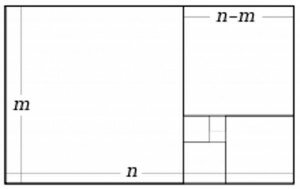Depicted here, the Golden Mean Ratio, and in the geometries thought to be applied by Thutmose, the Egyptian Court Sculptor, in the creation of Nefertiti’s Bust:

n is to m, as m is to n − m,

or, algebraically,

n            =         m

_____            ______

m                     n – m

A more clear example of the pattern he believed Her Bust was created upon, the Fibonacci Sequence, can more easily be seen in examining nature’s pattern in pine cones, and seashells.  This ratio is also the most efficient mathematical equation for trees absorbing the most amount of sustenance from the sun’s rays, hence growing in a spiral.  In the same way, today, we can apply solar panels onto rooftops mimicking this pattern to most efficiently absorb the sun’s rays.  For further discussion on Fibonacci Sequence, visit here:  http://www.math-lessons.ca/fibonacci-sequence.html/

See in the photo at the top, the chamomile spiral, as well as in this link on “fibonacci-sequence” showing the pattern.  Adding two consecutive numbers from the sequence to equal the next one following, the basic mathematical sequence looks like this:

0,         1,            1,            2,            3,            5,            8,            13,            21,            34,            55,            89,            144,            233,            377, etc., etc.

That is:

0+1=1

1+1=2

2+1 (the “number” before)=3

3+2 (the number before)=5

5+3=8

8+5=13

13+8=21

34+21=55

55+34=89

89+34=144

144+233=377

etc., etc., etc.

Whereas the fibonacci sequence, cannot technically be exacted in real time, (similarly, there is no real exact end found as of yet to pi’s “3.14159265359…..”), mathematicians drew swirling squares around the spiral in the attempt to exact the formula – basically giving the left side of the human brain a way of understanding the right-brained infinite spiral, being that the left side of the brain requires finiteness / exactness to feel satisfied, shall we say.

What kind of intriguing application can your class find to prove that math is interesting and fun?

We wonder if the geometries of Nefertiti’s Bust were also the same geometries of Nefertiti’s real-life human head.  The skulls of Ancient Egyptians, as well as those of Ancient Greece and other cultures, have definitely been shown to have geometrically perfect structures.  How can that be?

For samples of our Fun Learning Math Games, feel free to visit here:

http://butterflybooks.ca/geometry-games/

http://butterflybooks.ca/math-activities/

## Torus Math – The Doughnut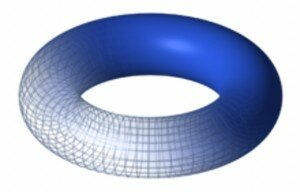Welcome Back to Math Class!  We trust all had a wonder-filled summer filled with fun spherical geometric shapes and bursting color!  Our Back-to-school math topic this autumn is about the math magic of the Torus. A torus is: the area and volume of, basically, a doughnut shape, shown here:
http://en.wikipedia.org/wiki/File:Torus.png
The author of harmonic resolution; The Portacle, describes that the torus’ special form has been used to describe and/or represent a number of things in our “real” actual material world, as well as, our “imaginary” potential one; and is defined by two parameters: the radius of the torus (that is, the radius of the torus’s defining circle, measured from the origin) and the radius of the tube (the perpendicular distance from the defining circle to the surface of the torus). These are R and r, respectively (R > r).   -In this link, there is a Great fluid-moving image of a spinning torus, about 4/5th down the page.)  Wikipedia has a diagram of the torus as being the product of 2 circles, one sideways inside the other: See image to the left here: A torus is the product of two circles, in this case the red circle is swept around the axis defining the pink circle. R is the radius of the pink circle, r is the radius of the red one; Image drawn August 28, 2014: http://en.wikipedia.org/wiki/File:Torus_cycles.svgSome say that Earth is neither flat or round, but spherical, as in that of a doughnut toroid or even an apple, curving down and curving upward at the North and South poles.  One image we find most interesting is just under the blue torus image, and it is a mutable image of a ring torus turning itself into a horn torus, and this is one of the images that mathematicians today are calculating that Earth is most closer to as a realistic geometric shape, than was once thought.  Earth, in this respect is today referred to as the tube torus, a shape that occurs often in Nature.  If you click on the link, you can see just to the right under the blue torus, a better mental picture of our reference: As the distance to the axis of revolution decreases, the ring torus becomes a horn torus, then a spindle torus, and finally degenerates into a sphere; www.wikipedia.org/wiki/torus.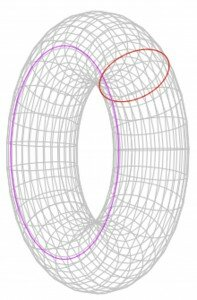http://en.wikipedia.org/wiki/File:Torus.png
(There are several on-line sources of the manifolds, 3 of which quote the same information:  Joyce, Wikipedia and Harmonic Resolution.com, all denoting that manifolds are today, a major branch of geometric study, a curved space of some dimension. For example, the surface of a sphere, and the torus (the surface of a doughnut), are both 2-dimensional flat-surface – perceived manifolds. The manifold itself is the background for some mathematical object defined upon it, as a canvas is the background for an oil painting. This kind of geometry, although very abstract, is closer to the real world than you might think. Einstein’s theory of General Relativity describes the Universe – the whole of space and time – as a 4-dimensional manifold.  Wikipedia has wonderful moving images of the manifold turning into the torus and back into a manifold flat surface again, and is worth having a gander for a more picturesque idea of what these terms are in Math, under the subtitle “ Flat Torus“: http://en.wikipedia.org/wiki/Torus: http://en.wikipedia.org/wiki/File:Torus_from_rectangle.gif

Dominic Joyce, Universty of Oxford, (www. maths.ox. ac.uk/people/profiles/dominic.joyce) iterates that today’s popular geometric math is in manifolds, no longer Euclid’s triangles and circles, and says that popularity went out with the Arc!  We think he is referring to Noah’s Arc, which many would probably disagree about Noah Arc’s popularity because of all the beautyful animals on the Arc!  But that is digressing….Joyce says that, Space itself is not flat, but curved. The curvature of space is responsible for gravity….and that, Everything in the universe – light, subatomic particles, pizzas, and even, yourself – is described in terms of a geometrical structure on the space-time 4-manifold.  Joyce says that, according to some physicists, the universe is now considered to be a 12 (or 13, 15, or 17, by some!) dimensional nested manifold.

In “superstring” physics, the torus is known as the “perfect” shape…but String Theory we can discuss on another day!

For more fun and interesting Learning Math Games, you can visit us here:

http://www.math-lessons.ca/activities/Geometry.html

http://www.math-lessons.ca/activities/FractionsBoard5.html

http://www.math-lessons.ca/timestables/times-tables.html

http://www.math-lessons.ca/activities/FractionsBoard4.html

## Perimeter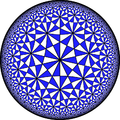The perimeter of a shape is the distance around the sides of the shape. Calculating and distinguishing characteristics of shapes is an important concept in Geometry. Elementary school students should be familiar with both 2-D (square, triangle, rectangle, circle) and 3-D shapes (cube, sphere, cylinder, pyramid, cone). Kindergarteners and first graders should be able to identify the shape by name, whereas 2nd and 3rd graders should be able to calculate the perimeter, and 4th and 5th graders should be well versed in area and volume calculations.

In this activity, students will explore the concept of perimeter and how to calculate the perimeter of regular polygons.

Materials:

Measuring tape

White board and dry erase markers

Note: If your classroom is not perfectly square or rectangular, consider drawing a classroom layout, on the white board that is easy to calculate the perimeter. For more advanced students, challenge them to find the perimeter of their irregularly shaped classroom.

Instructions

1. Have the students measure the dimensions of the classroom (to the nearest foot), drawing a diagram of the classroom.
2. As the students measure the classroom, either have them recreate the classroom on paper, or on the white board in the front of the classroom.
3. Explain to the class that they are calculating the perimeter of the classroom. You may consider saying, “The perimeter are the edges of the classroom that we are measuring. The perimeter tells you the distance around the outside of a shape. The classroom is shaped like a rectangle (or a square), so when do you think it would be helpful to know the distance around the classroom? Yes, if we were going to decorate the classroom with a ribbon, we would need to know the length of the sides of the classroom. The perimeter is an easy calculation to find, just by adding the length of each side together.”
4. Have the students calculate the perimeter of the classroom using their diagrams.

You can extend this activity and encourage the students to measure a room in their home and determine the perimeter of the room. This can also be done measuring the perimeter of the outside playground at the school, or the perimeter of the school’s desks.

For more ideas about how to teach young students about perimeter and distance of shapes, visit: http://www.scholastic.com/teachers/top-teaching/2012/12/10-hands-strategies-teaching-area-and-perimeter and http://www.watchknowlearn.org/Video.aspx?VideoID=32320&CategoryID=3337.

or more fun and interesting Learning Math Games, you can visit us here:
http://www.math-lessons.ca/activities/FractionsBoard5.html
http://www.math-lessons.ca/timestables/times-tables.html
http://www.math-lessons.ca/activities/FractionsBoard4.html

## Probability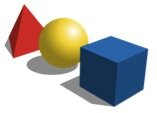What are the chances that you can flip heads or tails? Probability can be a fun guessing game and is an interactive Math concept that can be simple to introduce to elementary school students. Probability can tell you how likely or unlikely an outcome is, which can give you mathematical information to help you make a decision. If you have a 0.00001% chance of finding gold in your backyard, are you going to start digging for gold? (Shapes; downloaded Feb. 7, 2014;  http://commons.wikimedia.org/wiki/File:Basic_shapes.svg)  Probably not, right? Students can begin to learn that outcomes can be numerically calculated and then can convey valuable information.

In this activity students will learn how to calculate and interpret probability.

Materials:

Large bag of assorted color candy

Small plastic bowls

*It is best if you know how many color of each candy is present in the bag. If this is not possible, estimate the approx. numbers of each.

Instructions

1. Assemble the class into small groups.
2. Give each group a small plastic bowl and 1-2 cups of candy in each group’s bowl.
3. Have the students count the total number of candies, the number of candies of each color and record the data on a piece of paper.
4. Explain to the students that the probability of something occurring is another way of saying what are the chances or the likelihood that an event will happen. You may consider saying, “We are going to calculate the probability of you getting a red colored candy from your bowl, if you picked the candy with your eyes closed. The probability is a number that helps you determine if something is likely to happen or not likely to happen. To find the probability of picking a red candy, we need two numbers. The number of red candies in your bowl and the total number of candies in the bowl. In this group, they have 2 red candies and 31 total candies. So the probability would be 2/31, which is a very small number. So, if they were to close their eyes and choose a candy, it would most likely not be red.”
5. Invite students to calculate the probability of picking each color and allow time for them to pick candies at random to explore the concept of probability further.

For older students, extend the activity by practicing how to reduce fractions, for example a probability of 3/18 can be reduced to 1/6. There are lots of fun ways to teach probability. Check out these great online resources:  http://nrich.maths.org/probability and http://www.scholastic.com/browse/article.jsp?id=3756484.

For more fun and interesting Learning Math Games, you can visit us here:
http://www.math-lessons.ca/activities/FractionsBoard5.html
http://www.math-lessons.ca/timestables/times-tables.html
http://www.math-lessons.ca/activities/FractionsBoard4.html

## Writing Mathematical Ratios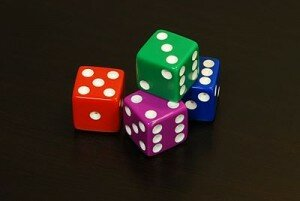Comparing numbers and quantities can be an advanced component of teaching Math, but can also be easily introduced to younger students in first or second grade. In Math, it is important to provide all students with mathematical vocabulary, like ratio or proportion. (Dice; downloaded Feb. 7, 2014; http://en.wikipedia.org/wiki/File:6sided_dice.jpg)  A ratio is nothing more than a comparison of two quantities and we use ratios even outside of the classroom. For example, when cooking, we may use 2 parts flour to 1 part sugar, that would be the ratio 2 : 1 or when mixing cleaning products, like 1/3 cup of bleach to 1 gallon of water. It is essential to explain complex terms like ratio or proportion in easy to understand language, and when possible using hands-on everyday items.

In this activity students will learn how to calculate and write a ratio.

Materials:

5 sealable bags, red/blue/green marbles or unit blocks (20 of each color), white board, dry erase markers *This is best done as a group activity with no more than 4 students per group. Prior to the activity, place a different amount of each marble color in each sealable bag. For example, Bag 1 may have 3 green, 4 blue, and 5 red. Label each bag with a number to better keep track of the bags and marbles used.

Instructions

1. Assemble the class into small groups.
2. Give each group a labeled bag of marbles.
3. Have the students count the total number of marbles in the bag and the number of marbles of each color and record the data on a piece of paper.
4. Have the groups rotate bags so that each group gets a new sealable bag and repeat steps 2 and 3.
5. Ask the students: “What did you notice about the number of marbles in each bag? What about the number of each color?”
6. Explain to the students that the number of each color marble can be written as a ratio or a comparison. You may consider saying, “A ratio is a number that compares to items together. For example, in Bag 1, there were 3 blue marbles and 5 red marbles, so we could compare or write a ratio of blue marbles to red marbles by writing 3 : 5.”

For older students, consider extending the activity by providing them with word problems involving ratios. Have them explore how ratios can be maintained by doubling or halving quantities. For example, you might ask, “if the marbles are to stay in a 3 : 1 ratio, how many marbles of each color would you need if you wanted to double the total number of marbles in Bag 3?”

Ratio activities can be a great tool to teach fractions in the classroom. For more creative math teaching tips, visit: http://nrich.maths.org/4825 and http://mathsnacks.com/teacher.php .

For more fun and interesting Learning Math Games, you can visit us here:
http://www.math-lessons.ca/activities/FractionsBoard5.html
http://www.math-lessons.ca/timestables/times-tables.html
http://www.math-lessons.ca/activities/FractionsBoard4.html
http://www.math-lessons.ca/index.html

## Order of Operations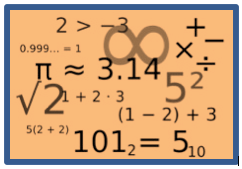Math is like a special type of language that has rules that must be followed. Understanding that the order of numbers and how they are combined is an important concept that elementary school students must master. Just as in everyday activities, like getting dressed, cooking, reading, the order of the steps matter. We would not put our shoes on before putting on socks, or read a book before opening it. Making this concept come to life for young students is easy to do using everyday analogies and hands-on activities.In this activity students will explore the mathematical order of operations.

Materials:

Several clothing items (coat, shirt, socks, hat, sunglasses, gloves)

Whiteboard and dry erase markers

Instructions

1. Invite a volunteer to come to the front of the classroom.
2. Provide them with the various clothing items and explain to the class that even in everyday tasks, like getting dressed, there is a particular order or “rules” that we follow.
3. Ask the student to put the items on (over top of their clothes to simulate getting dressed). As the student does, so point out what item went first, then next.
4. Acknowledge that the order of the hat and sunglasses did not matter. One could either put the sunglasses on first and then the hat or vice versa.
5. Explain how this relates to Math and the order of operations. You may consider saying, “Just like with getting dressed, Math has a special order to it. We do operations that are in parentheses first, then those that may involve exponents, then multiplication and division, then finally, addition and subtraction. However sometimes the order does not matter, like with the sunglasses and hat. When you are only left with addition and subtraction or multiplication and division, you do the operations as they appear from left to right.”
6. Write the following problem on the board: (3 x 5) – 60 ÷ 10 + 7 and walk students through the “order” of what has to be done first, then next, then last. If time permits, write additional problems on the whiteboard and have students work through the order of operations.

Additional Order of Operation Resources: As students graduate to more advanced math, it is essential that they be able to accurately manipulate and calculate values. This is especially true with solving equations or problem-solving. For more interactive ways to introduce and practice the order of operations, visit:

## Enjoy Making Bar Graphs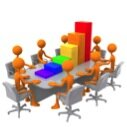Using graphs to represent data is an important feature of teaching math to elementary school students. Graphs come in many different shapes and sizes and can convey numerous types of information. As students progress through Math, they will encounter graphs in increasing complexity and will be asked to interpret data from graphs, draw conclusions from graphs, and even extrapolate information. Introducing graphing can be done through fun, interactive games that bring Math to life.  (photo: www.commons.wikimedia.org/wiki/File:3D_Bar_Graph_Meeting.jpg (10/27/13 7:15pm)

In this activity students will explore the how to collect and graph discrete data.

Materials:   Chart Paper, Markers

Instructions:

1. In the classroom, section off 4 distinct areas. Using corners of the classroom is the easiest way to do this.
2. Ask the students: “What is your favorite dessert? If you like cake, go to Corner #1; if you like cookies, go to Corner #2; if you like ice cream, go to Corner #3; if you like candy, go to Corner #4.
3. Allow time for students to decide which dessert they prefer and then record the number of students in each corner.
4. On the chart paper, have 4 columns, one for each dessert option. Write the number of students in the corresponding column.
5. Explain to the class that they just collected data on the type of dessert their classmates like. You may consider saying, “Data can be in the form of numbers or words, and in this case, we determined how many of you like each type of dessert. Next, we are going to do a graph, which is similar to a picture, showing the data we just collected.”
6. Create the axis of the graph, labeling the number of students on the vertical axis (y-axis) and the type of dessert on the horizontal axis (x-axis).
7. Mark the y-axis according to provide enough numbers to represent the numbers of students in each category. Draw in the bars to the corresponding number for each dessert type. For younger students, consider using stickers to represent the bars of the graph and have each student place a sticker in the dessert column they prefer.

Graphing is essential to building scientific knowledge and understanding as well as Math comprehension. For more interesting graphing activities, visit:

And for more of our Fun Learning Math Games, you can visit here:

www.math-lessons.ca/activities/index.html

www.math-lessons.ca/activities/FractionsBoard5.html

www.math-lessons.ca/activities/Geometry.html

http://www.literature-enrichment.com/

## Teaching Functions and Addends with Fun Machines!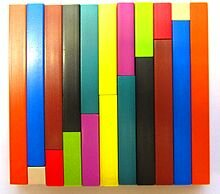This activity may be the most enjoyable way to introduce and practice functions. It can be adapted to meet the needs of a variety of grade levels.

Preparation: You will need a large box (large enough for a student to sit inside of it). Most appliance stores or big box stores will be happy to donate one. You can have the class decorate it (as a recess or free time activity) or you may choose to decorate it yourself. Turn the box upside down and cut out a “doorway” on one side of the box. Then cut two openings (similar to mailbox slots) on the front of the box and identify them as “in” and “out” slots. Once the “Magic Function Machine” is created, it can be used year after year.

Activity:

1)     Have a student sit inside the Magic Function Machine with all needed materials. You can use a variety of items such as counters, dry-erase boards, paper and pencil, craft sticks or any other item you can think of.

2)    The Teacher introduces the rule for the function machine. “This Magic Function Machine is so smart; it always adds 5 to any number you tell it. Who wants to try it out?”

3)    Choose a volunteer to come to the machine and input a number. They can write in on paper, write it on a whiteboard, count out the correct number of counters, etc. They put this number into the “in” slot in the machine and announce to the class what number they gave the machine (“I am putting in 6 craft sticks.”)

4)    Students at their desks figure out what the machine should output while the student in the box does the same.

5)    Give the students time to complete the problem. Then, ask the machine to send the output. “I think we are ready. What is the answer Magic Function Machine?”

6)    The student inside the box sends the answer through the “out” slot. The student outside the box announces the results to the class. (“I put 6 craft sticks in, and the magic machine gave me 11 craft sticks back.”)

Variations:

1)    As students become more proficient, they can create the rule they would like the machine to follow.

2)    Practice missing addends (an important algebraic concept). Have the students figure out the rule rather than the output. (“I put in 7 and 10 came out. What rule is the magic machine using?”)

3)    The complexity of the problems can change based on the abilities of your students. It is simple to individualize this activity.

4)    The problems can vary based on concepts students are practicing (addition, subtraction, multiplication, division, 2-step problems, negative numbers, fractions, etc.)

## Learning Measurement in Elementary Math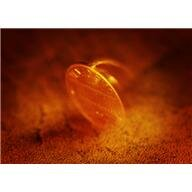Are you finished administering the same old paper and pencil tests to your students? Instead, why don’t you allow you students to demonstrate what they have learned? Here is a great way to assess your students’ learning with measurement units, using performance assessment.

We find that it is easiest to set the desks up in a circle so that students can move clockwise and there is no confusion as to where to move next. Use large index cards to number the problems and describe the task the student must complete while at that desk. A different problem is presented at each desk. Use a numbered index card to clearly indicate the problem number. (See Figure 1 for an example.)

Record The Value of The Five Coins

Students start at their own desk, recording the answer for that problem. All students will start on a different number. It may be helpful to provide young students a numbered table to record their answers. I find it is helpful to have students put a star on the number problem that is set up at their desk so they start recording answers in the correct box. (See figure 2 for an example).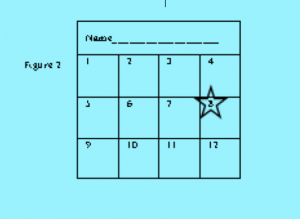Here is an example of the types of problems that can be used in this assessment. The materials needed at each desk are indicated in the parentheses.

Desk 1 – (coins) Record the value of the five coins.

Desk 2 – (clock) Record the time shown on the clock.

Desk 3 – (pencil, ruler) Measure the length of a pencil to the nearest half-inch.

Desk 4 – (calendar) Record the date of the second Wednesday in March.

Desk 5 – (apple, scale, gram weights) Find the weight of the apple to the nearest gram.

Desk 6 – (clock) Identify the time on the clock. What time was it 15 minutes earlier?

Desk 7 – (calendar) Today’s date is highlighted on the calendar. Marie’s birthday is in 12 days. What is the date of Marie’s birthday?

Desk 8 – (blackboard eraser, ruler) Measure the length of the eraser to the nearest centimeter.

Desk 9 – (clock) Charles started his homework at 3:45. He worked for 20 minutes. What time was it when he finished his homework?

Desk 10 – (1-inch squares and an outline of a large rectangle) Lay the squares on the rectangle to determine the area of the rectangle. Remember to label your answer with square inches.

For more fun and interesting Learning Math Games, you can visit us here:
http://www.math-lessons.ca/activities/FractionsBoard5.html
http://www.math-lessons.ca/timestables/times-tables.html
http://www.math-lessons.ca/activities/FractionsBoard4.html
http://www.math-lessons.ca/index.html

## Developing Number Theory and Fraction Concepts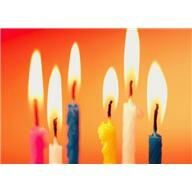Many students can begin to feel challenged in math in middle school. Students who have been good at, and have even enjoyed, math suddenly look to their teachers, friends or parents for assistance. Why does this happen? If you look at the concepts that are significant in middle school grades (fractions, decimals and integers), you find that these concepts appear to break all the rules their teachers have told them up to this point.

Typically, students are taught that when you multiply two numbers, the product is always larger. When you divide two numbers, the quotient is always smaller. However, these rules apply to whole numbers, not fractions. When you multiply two fractions, the resulting product may be smaller! When you divide two fractions, the quotient may be larger!  Many students become frustrated, confused and give up on math. As teachers, we need to make sure our students understand concepts, not just memorize rules about them. Students need time to explore and discuss real life examples of the concepts we are teaching. Below are some high-level tasks that allow students to explore number theory and fraction concepts. As with all tasks, students should represent their work in numbers, pictures and/or words. They should have time to communicate their thoughts and findings with others.

Topic: Factors and Multiples

Task: Max is making table favors for a party. The candles come in boxes of 15 and the candleholders come in boxes of 9. Max does not want any leftover candles or holders. What is the fewest number of candles and candleholders he needs without any leftover? How many boxes of each should he buy? Task: At a day camp, there are 12 girls and 18 boys. The camp counselors would like to split the campers into teams. However, they must follow these rules: 1) All campers must be on a team; nobody can be left out, 2) all teams must have the same number of campers, and 3) each team can only have all boys or all girls; no boys and girls can be on the same team. What is the greatest number of camperseach team could have?

Topic: Understanding Fractions

Task: In Penny’s Pet Shop,  of the pets were dogs,  of the pets were cats,  of the pets were birds and the rest were gerbils. There were 48 pets in all. How many of each type of pet were there? Task: Ms. Kinny has  tank of gas in her Volkswagen Beetle. Miss Jamison has  tank of gas in her Ford Mustang. Dr. Beck has  tank of gas in her Honda Accord. Mrs. Hughey has  tank of gas in her Toyota Prius. Without finding common denominators, list the women in order from the person who has the least amount of gas in her car to the person who has the greatest amount of gas in her car.

And for more of our Fun Learning Math Games, you can visit here:

http://www.math-lessons.ca/activities/index.html

http://www.math-lessons.ca/activities/Geometry.html